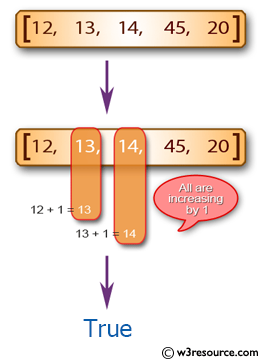﻿ Swift Array Programming Exercise: Test if a given array of integers contains three increasing adjacent numbers - w3resource# Swift Array Programming Exercises: Test if a given array of integers contains three increasing adjacent numbers

## Swift Array Programming: Exercise-37 with Solution

Write a Swift program to test if a given array of integers contains three increasing adjacent numbers.

Example: a) 3, 4, 5 b) 7, 8, 9

Pictorial Presentation:Sample Solution:

Swift Code:

``````func adjacent_numbers(array_nums: [Int]) -> Bool {
for x in 0..<array_nums.count-2 {
if array_nums[x] == array_nums[x+1] - 1 && array_nums[x+1] == array_nums[x+2] - 1
{
return true
}
}

return false
}

print(adjacent_numbers(array_nums: [1, 4, 5, 6, 2]))
print(adjacent_numbers(array_nums: [1, 2, 7, 4, 5]))
print(adjacent_numbers(array_nums: [-4, -3, -2, -1, 0]))
```
```

Sample Output:

```true
false
true
```

Swift Programming Code Editor:

Improve this sample solution and post your code through Disqus

What is the difficulty level of this exercise?

﻿